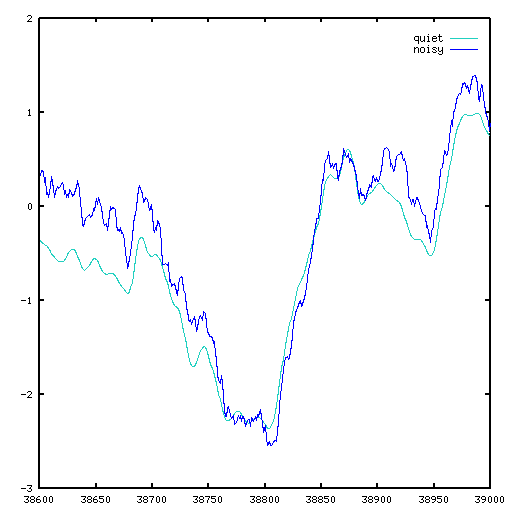# State Observers: Theory

Developing Models for Kalman Filters

In the last series of articles, we discussed the problem of obtaining a dynamic state transition model. To use the model, input values are applied to the model; it does whatever it does to update its hidden internal state variables, and as a consequence of this it is able to produce predictions of what the system output is expected to be.

The limitation of this approach is that it is somewhat divorced from reality. To understand what that means, let's perform two simulations using the model. In the first simulation, apply the original input sequence, and observe the sequence of output predictions. Then, for the second simulation, add about 4% zero-mean uniform random noise into each state variable after each update. The second simulation won't be an exact prediction of anything, but it will illustrate the kind of effects to expect.Once the noise has become integrated into the state of the model, some significant effects can accumulate. If noise produces stray effects of something like 20 percent or more, it becomes difficult to determine meaningful model parameter adjustments that produce any better results.

## Models and state estimation

The problem with operating the model as we have done previously is that it is a little divorced from reality. The model predictions are completely separate from what the system is actually doing. If the model predictions are actually proving to be quite wrong, the model has no way to know that. It can't see what hidden random disturbances are doing to the real system state.

So, suppose we formulate a different kind of problem. Assume that the system model is known and capable of making highly accurate predictions — provided that it has highly accurate estimates of the system state variables. In this alternative problem, the goal is to use all available information about the system inputs and outputs to produce the best possible estimate of the state variable values. This is called the state observer problem.

The state transition equations and the state observer equations are in some respects analogous to open loop and closed loop control strategies from control theory. In an open loop strategy, you know from past studies how your system is going to respond to a given input signal, so the best control strategy is to apply the known input signal that will take your system from its initial state to the target state. Does the system actually get there? Probably not, but close enough to make this strategy very useful. In a closed loop strategy, you observe the actual system output, compare it to what the system output should be, and based on this, apply a feedback adjustment to incrementally correct the discrepancy. The two strategies can be applied together to form a powerful combination.

## State observer equations

We are going to start getting a little more formal now. Observer equations are a set of equations that attempt to deduce from the system input and output sequences what the best estimated values of state variables are. As you can imagine, the relationship between the observer equations and state transition equations is close, and we want to take advantage of the known state transition equations as much as possible. The system state transition equations governing the linear system are:

${x}_{k+1}\phantom{\rule{1.0em}{0ex}}=\phantom{\rule{1.0em}{0ex}}A{x}_{k}\phantom{\rule{1.0em}{0ex}}+\phantom{\rule{1.0em}{0ex}}B{u}_{k}\phantom{\rule{1.0em}{0ex}}+\phantom{\rule{1.0em}{0ex}}{v}_{k}$

where

`x` is the system state vector, hidden, not directly observable
`u` is the vector of input values
`A` is the state transition matrix for determination of next state
`B` is the input coupling matrix routing input variables to changes in state
`v` is random unknown variation or disturbance
`k` is a time index, indicating current and next time instant

The associated output observation equations specify how hidden states produce observable output values.

${y}_{k}\phantom{\rule{1.0em}{0ex}}=\phantom{\rule{1.0em}{0ex}}C{x}_{k}\phantom{\rule{1.0em}{0ex}}+\phantom{\rule{1.0em}{0ex}}D{u}_{k}\phantom{\rule{1.0em}{0ex}}+\phantom{\rule{1.0em}{0ex}}{r}_{k}$

where

`y` is the vector of observed outputs
`C` is the observation matrix expressing relationship of outputs to state
`D` is the direct effects of inputs on outputs (mostly undesirable)
`w` is random unknown variation in the observation process

Though model matrices `A, B`, and `C`, are presumed known from the state transition equations, the state variable values and the random disturbance values are not known. The new state observer problem is to select observer matrices `E, F`, and `G` that define a new state observer equation dynamic system to be determined. All state variables of this system are completely observable, and they provide estimates of the values of the system state variables.

${z}_{k+1}\phantom{\rule{1.0em}{0ex}}=\phantom{\rule{1.0em}{0ex}}E{z}_{k}\phantom{\rule{1.0em}{0ex}}+\phantom{\rule{1.0em}{0ex}}F{y}_{k}\phantom{\rule{1.0em}{0ex}}+\phantom{\rule{1.0em}{0ex}}G{u}_{k}$

Contents of the "Developing Models for Kalman Filters" section of the ridgerat-tech.us website, including this page, are licensed under a Creative Commons Attribution-ShareAlike 4.0 International License unless otherwise specifically noted. For complete information about the terms of this license, see http://creativecommons.org/licenses/by-sa/4.0/. The license allows usage and derivative works for individual or commercial purposes under certain restrictions. For permissions beyond the scope of this license, see the contact information page for this site.